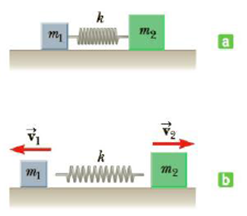# Two objects of masses m 1 = 0.56 kg m 2 = 0.88 kg are placed on a horizontal frictionless surface and a compressed spring of force constant k = 280 N/m is placed between them as in Figure P6.28a. Neglect the mass of the spring. The spring is not attached to either object and is compressed a distance of 9.8 cm. If the objects are released from rest, find the final velocity of each object as shown in Figure P6.28b. Figure P6.28### College Physics

11th Edition
Raymond A. Serway + 1 other
Publisher: Cengage Learning
ISBN: 9781305952300### College Physics

11th Edition
Raymond A. Serway + 1 other
Publisher: Cengage Learning
ISBN: 9781305952300

#### Solutions

Chapter
Section
Chapter 6, Problem 28P
Textbook Problem

## Two objects of masses m1 = 0.56 kg m2 = 0.88 kg are placed on a horizontal frictionless surface and a compressed spring of force constant k = 280 N/m is placed between them as in Figure P6.28a. Neglect the mass of the spring. The spring is not attached to either object and is compressed a distance of 9.8 cm. If the objects are released from rest, find the final velocity of each object as shown in Figure P6.28b.Figure P6.28

Expert Solution
To determine
The velocities of two objects.

### Explanation of Solution

Given Info: Mass of object 1 is m1=0.56 kg , mass of object 2 is m2=0.88 kg , force constant of spring is k=280 N/m , compressed distance of a spring is d=9.8 cm , initial velocities of two  objects are (v1&v2)i=0 and movement of object towards the right is taken as the positive direction.

Explanation:

Apply conservation of momentum to object1 and object2 system

(m1v1+m2v2)i=(m1v1+m2v2)f (1)

• m1 is the mass of the object 1.
• m2 is the mass of the object 2.
• v1 is the velocity of the object 1.
• v2 is the velocity of the object 1.

Use above equation as v1,i=0 and v2,i=0 rewrite for v2 .

(m1v1+m2v2)f=0m2v2=m1v1v2=(m1m2)v1 (2)

Apply conservation of energy to the system

KE1f+KE2f+PEf=KE1i+KE2i+PEi

• KE1i is the kinetic energy of object 1  initially at  rest
• KE2i is the kinetic energy of object 2 initially at rest
• KE1f is the kinetic energy of object 1 moves finally
• KE2f is the kinetic energy of object 2 moves finally
• PEi is the potential energy of the objects initially at rest
• PEf is the  potential energy of the objects moves finally

Substitute 0 for KE1i,KE2i,PEf in equation (2).

KE1f+KE2f=PEi (3)

Use 1/2m1v12 for KE1f , 1/2m2v22 for KE2f and 1/2kd2 for PEi .

12m1v12+12m2v22=12kd2m1v12+m2v22=kd2 (4)

• k is the spring constant

### Want to see the full answer?

Check out a sample textbook solution.See solution

### Want to see this answer and more?

Bartleby provides explanations to thousands of textbook problems written by our experts, many with advanced degrees!

See solution